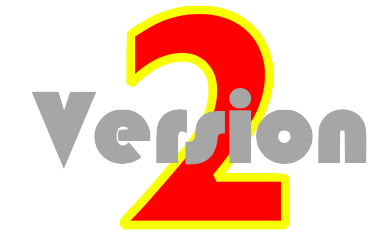### Newton's Laws of Motion

A Concept-Builder is an interactive questioning module that presents learners with carefully crafted questions that target various aspects of a concept. Each Concept Builder focuses the learner's attention upon a discrete learning outcome. Questions target that outcome from a variety of angles using multiple difficulty levels or varying activities. Learners ponder the given information and answer questions in the hopes of earning Stars and Trophies. These Stars (for answering questions) and Trophies (for completing levels or activities) are displayed on the screen, allowing a teacher to track student progress. The following Concept Builders target concepts associated with Newton's Laws of Motion.

Balanced vs. Unbalanced ForcesLearning Goal:  To analyze a variety of representations of an object’s motion and to draw conclusions about the state of motion and the relative strength of the forces acting on the object.

Force and MotionLearning Goal:  To use an understanding of the effect of balanced and unbalanced forces upon the motion of an object in order to predict how an object will move under a certain set of force conditions.

Change of StateLearning Goal:  To identify the manner in which the individual forces must change in order for an object to change its state of motion.

Recognizing ForcesLearning Goal: To utilize an understanding of the various types of forces in order to analyze a given situation and to identify the presence or absence of a variety of force types.

Match That Free-Body DiagramLearning Goal: To identify the free-body diagram (FBD) that is consistent with a given physical situation.

Normal Force Card SortLearning Goal: To analyze a physical situation in order to determine the relative strength of the normal force experienced by an object.

Which One Doesn't Belong? Force and MotionLearning Goal: To interpret various representations of an object’s motion in order to relate the relative strength of the forces that act upon the object to the subsequent motion of the object.

Newton's Second Law - Equations as Guides to ThinkingLearning Goal: To use Newton’s second law to predict the effect of varying net force and varying mass upon the acceleration of an object.

Net Force (and Acceleration) Ranking TasksLearning Goal: To use an understanding of Net Force and Newton’s Second Law to rank objects according to their net force and their acceleration.

Air Resistance and SkydivingLearning Goal: To combine an understanding of the factors affecting air resistance with an application of Newton’s second law and kinematic concepts in order to analyze a skydiver’s motion.

Fnet = m•aLearning Goal: To use Newton's second law equation to determine the individual force values required to cause a specified acceleration.

Solve It! with Newton's Second LawLearning Goal: To use the Fnet = m•a equation to analyze situations involving unbalanced forces and accelerations.